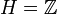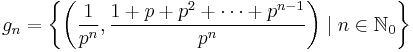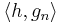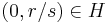# Pure subgroup of torsion-free abelian group not implies direct factor

This article gives the statement and possibly, proof, of a non-implication relation between two subgroup properties. That is, it states that every subgroup satisfying the first subgroup property (i.e., pure subgroup of torsion-free abelian group) need not satisfy the second subgroup property (i.e., direct factor)
View a complete list of subgroup property non-implications | View a complete list of subgroup property implications
Get more facts about pure subgroup of torsion-free abelian group|Get more facts about direct factor
EXPLORE EXAMPLES YOURSELF: View examples of subgroups satisfying property pure subgroup of torsion-free abelian group but not direct factor|View examples of subgroups satisfying property pure subgroup of torsion-free abelian group and direct factor

## Statement

### Statement for pure subgroups

It is possible to have the following:

•$G$ is a torsion-free abelian group
•$H$ is a pure subgroup of$G$ (this means that for any$h \in H$ and$n \in \mathbb{N}$ such that$nx = h$ has a solution for$x \in G$, there is a solution for$x \in H$).
•$H$ is not a direct factor of$G$.

### Statement for local powering-invariant subgroups

It is possible to have the following:

•$G$ is a torsion-free abelian group
•$H$ is a local powering-invariant subgroup of$G$ (this means that for any$h \in H$ and$n \in \mathbb{N}$ such that$nx = h$ has a unique solution for$x \in G$, that unique solution is in$H$).
•$H$ is not a direct factor of$G$.

## Proof

Example where$H = \mathbb{Z}$,$G/H = \mathbb{Z}[p^{-1}]$ for some prime number$p$:

Define$G$ as the subgroup of$\mathbb{Z}[1/p] \times \mathbb{Z}[1/p]$ with the following countable generating set:$g_n = \left \{ \left(\frac{1}{p^n}, \frac{1 + p + p^2 + \dots + p^{n-1}}{p^n} \right) \mid n \in \mathbb{N}_0 \right \}$

Explicitly, the generators are:$(1,0), \left(\frac{1}{p}, \frac{1}{p} \right), \left(\frac{1}{p^2}, \frac{1 + p}{p^2}\right), \left(\frac{1}{p^3}, \frac{1 + p + p^2}{p^3} \right), \dots$

We note that this generating set has the property that, for all$n \ge 1$:$pg_{n -1} - g_n = (0,1)$

Say$h = (0,1)$ and take$H$ as the subgroup$\langle h \rangle = \{ (0,a) \mid a \in \mathbb{Z} \}$.

We therefore get, for all$n \ge 1$:$\langle g_0, g_1, \dots, g_n \rangle = \langle h, g_n \rangle$

###$G$ is a torsion-free abelian group

This is obvious from it being a subgroup of$\mathbb{Q} \times \mathbb{Q}$.

### The element$(0, 1/p)$ is not in$G$

If$(0, 1/p) \in G$, it must be in the subgroup generated by finitely many generators:$\langle g_0, g_1, \dots, g_n \rangle$

As shown above, therefore, it must be in the subgroup:$\langle h, g_n \rangle$

The image modulo$\mathbb{Z} \times \mathbb{Z}$ is a cyclic group of order$p^n$. It's easy to see that there is no element in this image that equals the image of$(0, 1/p)$; in fact, forcing the first coordinate to map to zero in the quotient also forces the second coordinate to map to zero.

###$H$ is a pure subgroup

For this, it suffices to show that any element of the form$\left(0, \frac{r}{s} \right)$ that is in$G$ is also in$H$. First, if$r/s$ is reduced,$s$ must be a power of$p$, say$p^k$, and$r$ relatively prime to$p$. Next, the existence of$(0,r/s) = (0,r/p^k) \in G$ would force the existence of$(0,1/s) = (0,1/p^k) \in G$. If$k \ge 1$, this forces$1/p \in G$, which we saw is false from above. Thus,$k = 0$, so$s = 1$, and therefore the element$(0, r/s) \in H$.

### There is no complement to$H$ in$G$, so$H$ is not a direct factor

The quotient group$G/H$ is isomorphic to the first coordinate projection, which is$\mathbb{Z}[1/p]$, but there is no element in$G$ that has arbitrarily large$p^{th}$ power roots.